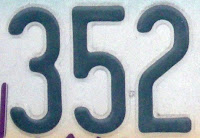## Monday, September 27, 2010

### 352

352 = 25 x 11.

352 has a unique representation as a sum of three squares: 352 = 82 + 122 + 122.

352 is the maximum number of volumes into which 11 spheres can divide space.

352 is the maximum number of regions into which 26 lines divide a plane.

352 is the only number consisting of three distinct prime digits such that the first and third equal the third raised to the power of the second (32 = 25).352 is a telephone area code for a region around Gainesville, Florida.